## teaching and educationJOURNAL OFAPPLIEDCRYSTALLOGRAPHY
ISSN: 1600-5767
Volume 33| Part 5| October 2000| Pages 1295-1301

## The number of good reflections in a powder pattern

aISIS facility, Rutherford Appleton Laboratory, Chilton, Oxon OX11 0QX, England
*Correspondence e-mail: dss@isise.rl.ac.uk

(Received 7 April 2000; accepted 26 June 2000)

The number of statistically independent' observations in a powder diffraction pattern has been the topic of some discussion over the past few years. It is argued that while this notion is tantalizing, it is illusory in principle, and it is suggested that a more appropriate measure of the quality of the data is the number of good' reflections. By way of explanation, a tutorial on the underlying concepts of correlation and covariance, which are a surprisingly common source of confusion, is provided. A practical procedure for implementing the theoretical ideas is proposed.

### 1. Introduction

In an ideal world, the vast majority of diffraction experiments would be performed on single-crystal samples rather than powdered ones, since experience has taught us that more detailed structural information can usually be inferred from the former. Although practical considerations can make the use of powder data unavoidable, it sometimes comes down to a matter of judgement: it may be possible to grow a sufficiently large single crystal, but only with considerable difficulty; is it worth the effort, or will a powdered sample suffice?

Such questions naturally prompt us to think about how the information content' in a powder diffraction pattern could be quantified, particularly with a view to comparing it with a comparable set of single-crystal data. Indeed, a quality measure of this type might also be useful for assessing the expected merit of any proposed change in the experimental procedure. The easiest way of addressing this problem is to consider the reliability with which the intensities of the structure factors, or the areas under the Bragg peaks, can be estimated from the data. This approach has the advantage of generality, in that it is largely independent of the details of the structure being studied, but the corresponding drawback that any resultant figure of merit' can only serve as a broad guide for a given specific situation.

An intuitive argument, which forms the basis of an algorithm for quantifying the impoverishment of powder data, proposed by Altomare et al. (1995), is outlined and discussed in §2. An account of the elementary concepts of correlation and covariance, which are central to the reasoning in this paper, but often a source of confusion for students and professionals alike, is given in §3; familiarity with basic science mathematics (Sivia & Rawlings, 1999), such as linear algebra and calculus, is assumed. This leads us to suggest, in §4, that it is better to think in terms of the number of good' reflections in a powder pattern rather than statistically independent' ones; a practical procedure for implementing the theoretical ideas is put forward and discussed with reference to a closely related proposal by David (1999).

### 2. An intuitive argument

If a certain region of a powder pattern consists of two well separated Bragg peaks, pertaining to two distinct reflections, then we can say that it contains exactly two independent pieces of intensity information; let us denote this by Ni = 2 and call the associated integrated intensities I1 and I2. A complete overlap, on the other hand, reduces Ni to unity because then only the sum I1 + I2 can be extracted reliably. For the general case of partial overlap, there will be some intermediate degree of correlation between the extracted intensities; this situation can be quantified by ascribing a suitable value to Ni that lies somewhere between 1 and 2. If this idea is generalized to deal with multiple overlaps and applied to the whole powder pattern, then Ni can be regarded as a measure of the equivalent number of statistically independent reflections inherent in the data; as such, it can serve as a useful guide for assessing the chances of successfully solving, or refining, a crystal structure. This intuitively reasonable argument forms the basis of a proposal by Altomare et al. (1995) for an algorithm for estimating Ni; while their suggested procedure has many appealing qualities, it also has some important shortcomings.

The most striking feature of the aforementioned proposal, which is based solely on the amount of overlap between Bragg peaks, is its insensitivity towards the quality of the data. This seems quite odd since the intensities of even highly correlated reflections can, in principle, be estimated to any arbitrary accuracy, with enough counting statistics, as long as the overlap is not complete; in practice, a limit is eventually set by how well the experimental parameters (such as the peak profile) have been modelled and calibrated. The point is illustrated in Fig. 1by computer-generated data from two closely spaced Lorentzian peaks, of full width at half-maximum (FWHM) 2 units, which are subject to a flat background signal and Poisson noise. Assuming that the profile and positions of the peaks are known, a least-squares analysis of the (X-ray or neutron) counts in Fig. 1(a) yields the following estimates for their intensities: I1 = 10.06 ± 0.50 and I2 = 6.47 ± 0.46. A similar analysis of Fig. 1(b) gives: I1 = 9.97 ± 0.07 and I2 = 7.12 ± 0.07. As expected, therefore, a 50-fold increase in the number of counts reduces the 1 − σ error bars by a factor of seven. This improvement is not reflected in the Altomare et al. statistic, however, which has a value of Ni = 1.50 for both cases.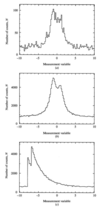Figure 1 Computer-generated data from two closely spaced peaks, of known shape and location, subject to a uniform background signal and Poisson noise. (a) and (b) are for the same Lorentzian peaks, but with different counting times; (c) pertains to a sharp-edged exponential, resembling a somewhat exaggerated version of the situation encountered at a pulsed-neutron source.

An even more disconcerting phenomenon is observed when the profile of the Bragg peaks is highly asymmetric. This is illustrated in Fig. 1(c), where the peak shape is a sharp-edged exponential (with FWHM = 2) rather than a Lorentzian, and resembles (a slightly exaggerated version of) the situation encountered at a pulsed-neutron source. In this case, a least-squares analysis yields I1 = 10.04 ± 0.10 and I2 = 7.00 ± 0.10, while the Altomare et al. statistic has a value of Ni = 1.00 (because the contribution to the signal from the peak on the right is always less than that from the one on the left, albeit only just so). Given that two signals can easily be distinguished by eye, the latter clearly fails to take adequate account of the shape of the Bragg peaks.

### 3. Correlations and covariance

In order to understand and overcome the difficulties resulting from the intuitive argument of the previous section, we need to take a step back and focus more carefully on exactly what we are trying to do. The most direct link between diffraction data and crystal structure is through the measurement of the intensities of the structure factors: the better we can estimate the intensities, the less uncertainty there will be in the inferred structure. Therefore, we need to consider how well the diffraction data constrain the range of intensities that yield reasonable agreement with them. How can we assess this quantitatively?

The quality of the fit to experimental measurements is often specified through the use of a χ2 statistic: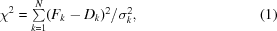where Dk is the kth datum, with error bar σk, and Fk is the corresponding prediction given by a proposed model. If the measurements pertain to counting statistics, then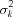is usually set to Dk or Fk to reflect the assignment of a Poisson uncertainty (Sivia, 1996). In the simplest situation, if we had a model defined by a single unknown parameter, x say, and its value was sufficient to evaluate the {Fk}, then a plot of χ2 versus x would give a graphical indication of the range of x values that yield reasonable agreement with the data. Or, rather, this would be so if we could interpret χ2 in a probabilistic sense.

The χ2 statistic is, in fact, related to the likelihood function, or the probability of the data given the model, through an exponential: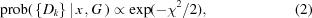where the G in the conditioning statement represents all the relevant background information and analysis assumptions, such as a knowledge of the experimental setup. For example, the use of equation (2) reflects the common assertion that each datum is subject to independent additive Gaussian noise of known variance. If it was thought that the error bars were uncertain to within a global multiplicative constant, β say, so that all the σk should really be βσk, as might happen if the data were only known to be proportional to the number of counts rather than being on an absolute integer scale, then it can be shown that the likelihood is proportional to a power of χ2 instead of its exponential (Sivia, 1996):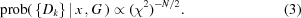Whatever the assignment of the likelihood function, it is usually convenient if the resultant plot of prob({Dk}|x,G) versus x can be summarized by a best-fit value, x0, and a number, , that indicates the range of the deviation of x from x0 which gives a reasonable agreement with the measurements. Such a specification of x = x0 ± is, in turn, most useful if the likelihood function can be approximated by a Gaussian: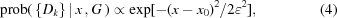a form that is readily ascertained if L = ln[prob({Dk}|x,G)] is expanded as a quadratic Taylor series about its maximum, so that x0 and are given by the first and second derivatives of L: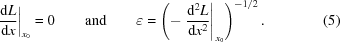For x0 to be a maximum, of course, we also need to ensure that d2L/dx2 < 0. Although equations (4)and (5)are only exact for the likelihood assignment of equation (2), when L = −χ2/2, and even then only when x is related linearly to the {Fk}, they do usually provide a good approximation. A better experimental design is simply one that reduces , for a given amount of time, money, effort, or whatever.

To avoid any subsequent confusion, we should emphasize that x0 does not necessarily represent our best estimate of x: it is merely the value of x which makes the data most probable. Despite the sense that is conjured up by the technical term for x0 as the maximum-likelihood estimate, our inference about x is encapsulated in the posterior probability, prob(x|{Dk},G), rather than the object of our current attention, prob({Dk}|x,G). These two entities are related, however, by the Bayes theorem,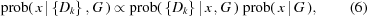but are only strictly proportional to each other for a uniform assignment of the prior probability, prob(x|G) = constant for all x. An obvious exception to an unqualified equivalence arises when our prior knowledge tells us that x must be positive on physical grounds, so that prob(x|G) = 0 for x < 0; in that case, x0 cannot be the best estimate of x if x0 < 0. We leave a further discussion of this point, with reference to the intensities and amplitudes of structure factors, to Sivia & David (1994), and return to the central theme of the likelihood function, for it is this quantity which enshrines the constraints imposed by the data themselves on the possible value of x.

Having set the stage by considering the most elementary situation, in which there is only one unknown parameter, let us move on to the more realistic multivariate case. For the sake of clarity, we will highlight the salient points by focusing on a bivariate problem and then indicate its straightforward generalization. It is rather like meeting partial differentiation for the first time as undergraduates: there are important new concepts to master in going from functions of one variable to two, but nothing fundamentally different in the progression from two to many.

Suppose we are interested in inferring the values of two quantities, x and y say, from a pertinent set of data. For example, the areas of two closely spaced Bragg peaks in an isolated region of a powder diffraction pattern where, for the moment, we will take it as given (in G) that the locations, peak shapes and background are known. Then, the information about x and y inherent in the experimental measurements is encapsulated in the two-dimensional likelihood function, prob({Dk}|x,y,G). A second-order Taylor series expansion of L = ln[prob({Dk}|x,y,G)] now leads to the approximation of the likelihood function as a bivariate Gaussian: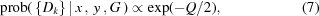where the scalar quantity Q is given by the quadratic form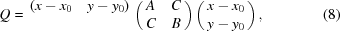with the best-fit estimates of x and y, x0 and y0, being defined by the condition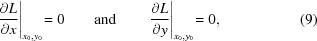and the elements of the symmetric 2 × 2 matrix, A, B and C, being given by the second partial derivatives of −L: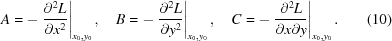To ensure that the stationary point at (x0, y0) is a maximum, we also need A > 0, B > 0 and AB > C2. The easiest way of visualizing the constraints imposed by the experimental measurements on the values of x and y is to consider the contours of constant Q, in equation (8), in a two-dimensional xy graph; these represent lines of equal likelihood, with the probability of the data being higher for smaller values of Q. The locus of Q = constant is, in fact, an ellipse centred on x = x0 and y = y0; its orientation and size are determined by the second-derivative coefficients in equation (10).

Four likelihood plots are shown in Fig. 2: (a) is for the case of the data in Fig. 1(a); (b) and (c) are for equivalent sets of measurements where the peaks are twice as far apart and twice as close together, respectively; (d) is for the data in Fig. 1(b). As the separation between the two peaks is reduced, the likelihood ellipses become increasingly skew and elongated with respect to the I1 and I2 axes; this is an indication of the correlation, or the difficulty in the disentanglement of the two intensities, that is inherent on the basis of the data being analysed. A longer counting time for the diffraction spectrum is helpful in that it gives rise to a more compact likelihood function and leads to a tighter constraint on the range of intensity values that yield reasonable agreement with the measurements; this shrinkage is often proportional to the square-root of the counting time.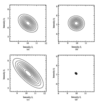Figure 2 The two-dimensional likelihood plots for the areas under the two peaks, or Bragg intensities. (a) is for the data in Fig. 1(a); (b) and (c) are for equivalent sets of measurements where the peaks are twice as far apart and twice as close together, respectively; (d) is for the data in Fig. 1(b).

The features illustrated in the examples above are common to many multiparameter estimation problems, so let us continue our discussion in terms of our generic xy notation. When faced with the quadratic form of equation (8), its analysis can always be made algebraically simpler by transforming from the original xy coordinates to a new set of basis vectors, X and Y, that lie along the principal axes of the ellipse: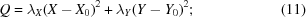a process called diagonalization. As such, the constants λX and λY, and the directions X and Y are the eigenvalues and eigenvectors of the 2 × 2 real symmetric matrix in equation (8). That is to say, they are given by the two solutions of the eigenvalue equation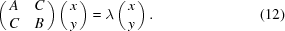The substitution of Q from equation (11) into equation (7), and a subsequent comparison with equation (4), leads to the conclusion that the information inherent in the data with regard to x and y can be summarized by the statement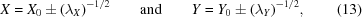where X and Y pertain to the two linear combinations of the original parameters generated by the components of the eigenvectors X and Y; explicitly, if X = (a, b) and Y = (c, d), then X = ax + by and Y = cx + dy. The reformulation of the problem in terms of X and Y is useful because they are the two quantities, related to x and y, that are independently constrained by the measurements: the likelihood function with respect to X is the same no matter what the assumed value of Y, and vice versa.

By contrast to X and Y, the equivalent specification of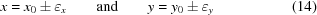would not generally be enough to capture all the salient information in the data. For example, the uncertainties x and y are given by (Sivia, 1996)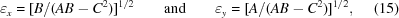and become infinitely large as C2AB; for our powder pattern, this would be the case when the two peaks became coincident. While equation (14)then correctly warns us that neither x nor y can be determined from the current data alone with any degree of reliability, it fails to tell us that they must satisfy a joint condition reasonably well in order to deliver agreement with the measurements; for the overlapping peaks, it would be a constraint on the sum of the two intensities (I1 + I2, or x + y). The point is that the permissible values of x and y are not independent of each other, and this additional information is conveyed through the covariance factor: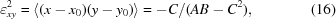where the angle brackets represent an expectation. If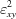> 0, then x and y are both likely to be underestimated or overestimated; a negative covariance indicates that an underestimate of one is expected to be accompanied by an overestimate of the other. The level of this type of interdependence is often given in terms of a correlation coefficient: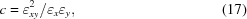where −1 ≤ c ≤ 1; complete correlation, or anticorrelation, is marked by c = ±1, whereas independence corresponds to c = 0.

Whether we think in terms of eigenproperties or covariance is largely a matter of personal preference, for they both reflect the constraints imposed by the data. Nevertheless, we will shortly see that the former offers some advantages in providing a straightforward quantification of the quality of the measurements. Before that, however, let us consider one further aspect of our two-peak example that will both aid a better understanding of correlations and indicate how our idealized situation can be relaxed towards a more realistic one.

Fig. 3shows data for two well separated Bragg peaks and the resulting likelihood function for their underlying areas. At first sight, this looks very strange: we would have expected the principal directions of the ellipse to lie along the axes of the intensities, thereby reflecting the independence of I1 and I2. The skewness arises from the fact that the analysis actually only assumed that the background signal was uniform, or a constant b, but not that its value was given. As such, the fit to the data is a function of I1, I2 and b, with the spread of the likelihood ellipsoid being characterized by a 3 × 3 symmetric matrix whose elements consist of the second partial derivatives of −L. The inverse of this matrix (Sivia, 1996), along with the condition ∇L = 0, yields the summary I1 = 9.73 ± 0.42, I2 = 7.07 ± 0.38 and b = 1.85 ± 0.09, with correlation coefficients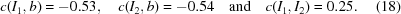While the peaks in Fig. 3(a) are far enough apart for I1 and I2 to be independent in principle, they become linked through a common uncertainty with regard to the value of b. Explicitly, with the limited set of measurements available, there is some difficulty in distinguishing the signal from the background in the neighbourhood of the peaks; the negative correlation of I1 and I2 with b, therefore, induces a positive one between the two intensities.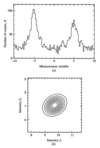Figure 3 (a) Computer-generated data from two well separated Lorentzian peaks, subject to a uniform background signal and Poisson noise. (b) The corresponding likelihood plot for the areas of the peaks.

The important thing about the illustration above is that it shows how the uncertainties in the uninteresting, but necessary, parameters in our model automatically filter through the analysis, and are reflected in the covariance factors for the relevant quantities that are returned. The likelihood functions in Figs. 2and 3(b) all pertain to 2 × 2 matrices, as in equation (8), constructed from the purely intensity-related subset of elements of the full 3 × 3 covariance matrix. Specifically, in our xy notation,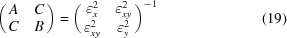and conforms with equations (8)and (16).

### 4. The number of good data

We have now reviewed the basic concepts, and analytical machinery, needed to address the question of the quality of diffraction data with regard to the intensities of the structure factors. The first thing to note is that the preceding discussion suggests that it is better to think in terms of the number of good' pieces of intensity information in a powder pattern, rather than the equivalent number of statistically independent' reflections. To see this, consider again the likelihood plots of Figs. 2and 3. The principal axes tell us the linear combinations of I1 and I2 which can be ascertained independently of each other: there are always two of them, irrespective of the separation of the peaks! The widths along these eigen-directions do vary with the degree of overlap, however, and indicate the reliability of the corresponding information. To be even more explicit, there are still two independent intensity-related quantities when the Bragg peaks become coincident: I1 + I2 and I1I2. It is just that the sum is well determined and can therefore be considered as being good, whereas the difference is completely unconstrained by the measurements (and so is bad, poor, or not useful). While the above might seem to be largely a case of semantics, we will shortly see that a failure to make a clear distinction can lead to practical consequences.

The generalization of the analysis procedure explained in the previous sectionis straightforward and essentially reduces to a Pawley refinement of the powder pattern (Pawley, 1981; David et al., 1992; David, 1999). Given a good initial estimate of the lattice constants, obtained from the locations of the low-order reflections, we will have a pretty good idea of the positions and number of Bragg peaks encompassed by the data; indeed, we can even lump together those which we expect to have little hope of separating to any extent as single compound entities and, thereby, avoid the risk of coming up against singular matrices. If there are M distinct intensity parameters in the problem, and m uninteresting ones (such as those pertaining to the background signal, peak shapes and lattice constants), then a Pawley-type analysis will return M + m best-fit values and a symmetric (M + m) × (M + m) covariance matrix. A reduced M × M matrix, Ω say, constructed from the purely intensity-related elements of the full Pawley covariance matrix, then characterizes the relevant information content of the data (within the usual context of the model assumptions and simplifying approximations used, of course). In formal terms,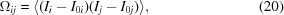for i and j = 1, 2, 3, …, M, and I0j is the best-fit intensity for the jth compound peak.

The quadratic form for the inverse of Ω in equation (20)yields an N-dimensional ellipsoid for the logarithm of the likelihood function, prob({Dk}|{Ij},G). Again, the eigenvectors of Ω−1 give the N different linear combinations of the {Ij} that can be ascertained independently of each other; the corresponding widths along the principal directions, which are inversely proportional to the square-root of the eigenvalues, as in equation (13), tell us how well these intensity factors are constrained by the measurements. The answer to the question of how to quantify the quality of diffraction data, therefore, lies in an examination of the eigenvalue spectrum, {λj}, of Ω−1: large λ values are associated with well determined (or good) quantities, whereas poorly constrained ones are indicated by λ → 0. The eigenvalue procedure that we are advocating is, in fact, nothing more than a classical singular value decomposition (SVD) analysis.

We should note in passing that Ω and Ω−1 share the same eigenvectors and have eigenvalues related simply by a reciprocal, as long as the matrices are not singular. This can always be ensured by a sufficiently conservative clumping together of closely spaced reflections (i.e. making M, and possibly m, smaller in the Pawley refinement).

In order to specify the number of well determined pieces of intensity information in a powder pattern, Ng, we need to define some form of threshold, ΔI, for discriminating between good and bad; in the simplest case, we could just add up the number of eigenvalues of Ω−1 that are bigger than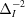. A more sophisticated variant on this counting procedure might be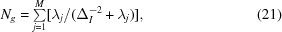where the contribution to the sum is unity if λjand zero if λj, so that a sharp cutoff is avoided. There is nothing fundamental about equation (21)[other than being analogous to a statistic that appears in classical maximum-entropy data analysis (Gull, 1989) which has a similar interpretation] or in any particular choice for ΔI. The issue is rather like that of having to decide which confidence level to use for quoting the results of a statistical analysis; the conventions of 70%, 90%, 95%, and so on, all tell part of the story but none can be considered the complete answer. The same is true here and, consequently, Ng will depend on ΔI since we are trying to convey the characteristics of a whole spectrum of M eigenvalues with a single number.

A useful measure for putting ΔI on a physically meaningful scale is probably the average value of the intensities, 〈I〉, as this is most likely to be estimated reliably. If ΔI = 0.85 for the data in Fig. 1, or 10% of the average intensity, for example, then equation (21)yields Ng = 1.53, 1.99 and 1.97 for Figs. 1(a), 1(b) and 1(c), respectively. As would be expected, a ten times more stringent requirement, ΔI = 0.085, reduces these Ng values to 0.07, 1.23 and 0.82, respectively. If the expectation value of the intensities varies appreciably across the diffraction pattern, due to a strong Debye–Waller or form-factor effect, then the analysis could be broken down into a series of more-or-less isolated regions which have their own local ΔI. This is equivalent to making the practical simplification that the covariance matrix is roughly block-diagonal, so that the intensities of the reflections are handled sequentially from contiguous chunks of the powder pattern.

Before concluding, we should mention a closely related analysis put forward by David (1999). The main difference from the proposal here is that David advocates the use of a matrix of correlation coefficients rather than the covariance matrix itself. The former, ω say, is derived from Ω by a straightforward generalization of equation (17),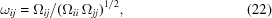and has the advantage that the eigenvalues of its inverse are automatically on an absolute scale; namely, isolated peaks have λ = 1. By associating values of λ less than unity with the degradation caused by correlation, it can be argued that a suitable measure of the effective number of independent peaks, Nind, is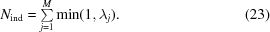While David points out that this statistic has many desirable properties, and demonstrates its usefulness with several examples, we still harbour a couple of concerns. The first is purely conceptual: if λ = 1 represents the ideal isolated case, and λ = 0 complete overlap, then, even though it is truncated in equation (23), what does λ > 1 mean? The second is more serious: since the counting-time aspect of the data cancels out in the ratio of equation (22), any procedure based on the eigenvalues of ω−1, instead of Ω−1, will be oblivious to the intrinsic quality of the measurements. Although the correlation-matrix approach of David is markedly superior to the Altomare algorithm, they share this common shortcoming.

### 5. Conclusions

We have discussed the information content of diffraction data in terms of the constraints they place on the intensities of structure factors. This involved a substantial account of the basic, but often poorly appreciated, concepts of correlation and covariance. It was argued that it is better to think in terms of the number of good pieces of intensity information in a powder pattern, rather than the effective number of independent reflections, with the relevant analysis reducing to a classical SVD exercise for the logarithm of the (marginal) likelihood function.

We conclude with the obvious, but important, remark that the information inherent in a powder pattern is only fully propagated to the space of structure-factor intensities if due account is taken of both the best-fit values and the covariance matrix. Either to ignore the latter completely, or only to consider its diagonal elements, is tantamount to assuming more, or less, respectively, than is justified by the measurements.

### Acknowledgements

Professor W. I. F. David is gratefully acknowledged for repeated encouragement with regard to the topic of this paper and for many related discussions.

### ReferencesJOURNAL OFAPPLIEDCRYSTALLOGRAPHY